xTwo-balloon experimentEncyclopedia
The two-balloon experiment is a simple experiment involving interconnected balloon
Balloon
A balloon is an inflatable flexible bag filled with a gas, such as helium, hydrogen, nitrous oxide, oxygen, or air. Modern balloons can be made from materials such as rubber, latex, polychloroprene, or a nylon fabric, while some early balloons were made of dried animal bladders, such as the pig...

s. It is used in physics classes as a demonstration of elasticity
Elasticity
Elasticity may refer to:*Elasticity , continuum mechanics of bodies that deform reversibly under stressNumerous uses are derived from this physical sense of the term, which is inherently mathematical, such as used in Engineering, Chemistry, Construction and variously in Economics:*Elasticity , the...

.

Two identical balloons are inflated to different diameters and connected together by means of a tube. The flow of air through the tube is controlled by a valve or clamp. The clamp is then released, allowing air to flow between the balloons. For many starting conditions, the smaller balloon then gets smaller and the balloon with the larger diameter inflates even more. This result is surprising, since most people assume that the two balloons will have equal sizes after exchanging air.

However, the outcome may vary depending on the initial sizes and elastic properties of the balloons involved. This makes even a system with idealised rubber balloons more complex than an equivalent with, say, soap bubbles. In addition, natural rubber exhibits hysteresis
Hysteresis
Hysteresis is the dependence of a system not just on its current environment but also on its past. This dependence arises because the system can be in more than one internal state. To predict its future evolution, either its internal state or its history must be known. If a given input alternately...

effects, and so what appears a simple experiment can produce extremely complex behaviour. The system has been modelled by a number of authors, for example to produce phase diagrams specifying under what conditions the small balloon can inflates the larger, or the other way round.

## Theoretical pressure curve

The key to understanding the behavior of the balloons is understanding how the pressure inside a balloon varies with the balloon's diameter. The simplest way to do this is to imagine that the balloon is made up of a large number of small rubber patches, and to analyze how the size of a patch is affected by the force acting on it.

The James-Guth
Eugene Guth
Eugene Guth was an American physicist who made contributions to polymer physics and to nuclear and solid state physics. He was awarded a Ph.D. in Theoretical Physics by the University of Vienna in 1928...

stress-strain relation
Stress-strain curve
During tensile testing of a material sample, the stress–strain curve is a graphical representation of the relationship between stress, derived from measuring the load applied on the sample, and strain, derived from measuring the deformation of the sample, i.e. elongation, compression, or distortion...

for a parallelepiped
Parallelepiped
In geometry, a parallelepiped is a three-dimensional figure formed by six parallelograms. By analogy, it relates to a parallelogram just as a cube relates to a square. In Euclidean geometry, its definition encompasses all four concepts...

of ideal rubber can be written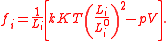Here,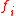is the externally applied force in the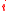direction,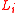is a linear dimension,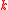is Boltzmann's constant,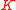is a constant related to the number of possible network configurations of the sample,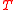is the absolute temperature,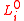is an unstretched dimension,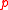is the internal (hydrostatic) pressure
Pressure
Pressure is the force per unit area applied in a direction perpendicular to the surface of an object. Gauge pressure is the pressure relative to the local atmospheric or ambient pressure.- Definition :...

, and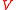is the volume of the sample. Thus, the force consists of two parts: the first one (caused by the polymer network) gives a tendency to contract, while the second gives a tendency to expand.

Suppose that the balloon is composed of many such interconnected patches, which deform in a similar way as the balloon expands. Because rubber strongly resists volume changes,the volumecan be considered constant. This allows the stress-strain relation to be written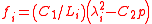where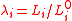is the relative extension. In the case of a thin-walled spherical shell, all the force which acts to stretch the rubber is directed tangentially to the surface. The radial force (i.e., the force acting to compress the shell wall) can therefore be set equal to zero, so that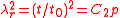where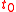and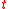refer to the initial and final thicknesses, respectively. For a balloon of radius, a fixed volume of rubber means that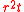is constant, or equivalently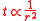hence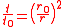and the radial force equation becomes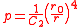The equation for the tangential force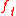(where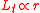) then becomes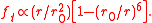Integrating the internal air pressure over one hemisphere of the balloon then gives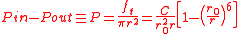where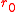is the balloon's uninflated radius.

This equation is plotted in the figure at left. The internal pressure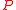reaches a maximum for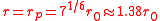and drops to zero asincreases. This behavior is well known to anyone who has blown up a balloon: a large force is required at the start, but after the balloon expands (to a radius larger than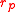), less force is needed for continued inflation.

## Why the larger balloon expands

When the valve is released, air will flow from the balloon at higher pressure to the balloon at lower pressure. The lower pressure balloon will expand. Figure 2 (above left) shows a typical initial configuration: the smaller balloon has the higher pressure. So, when the valve is opened, the smaller balloon pushes air into the larger balloon. It becomes smaller, and the larger balloon becomes larger. The air flow ceases when the two balloons have equal pressure, with one on the left branch of the pressure curve (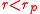) and one on the right branch (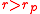).

Equilibria are also possible in which both balloons have the same size. If the total quantity of air in both balloons is less than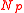, defined as the number of molecules in both balloons if they both sit at the peak of the pressure curve, then both balloons settle down to the left of the pressure peak with the same radius,. On the other hand, if the total number of molecules exceeds, the only possible equilibrium state is the one described above, with one balloon on the left of the peak and one on the right. Equilibria in which both balloons are on the right of the pressure peak also exist but are unstable.This is easy to verify by squeezing the air back and forth between two interconnected balloons.

## Non-ideal balloons

At large extensions, the pressure inside a natural rubber balloon once again goes up. This is due to a number of physical effects that were ignored in the James/Guth theory: crystallization
Strain crystallization
Strain crystallization is a phenomenon in which an initially amorphous solid material undergoes a phase transformation due to the application of strain. Strain crystallization occurs in natural rubber, and some other elastomers. The phenomenon has important effects on strength and fatigue...

, imperfect flexibility of the molecular chains, steric hindrances
Steric effects
Steric effects arise from the fact that each atom within a molecule occupies a certain amount of space. If atoms are brought too close together, there is an associated cost in energy due to overlapping electron clouds , and this may affect the molecule's preferred shape and reactivity.-Steric...

and the like. As a result, if the two balloons are initially very extended, other outcomes of the two-balloon experiment are possible.

Real balloons also experience hysteresis: the pressure depends not just on the balloon diameter, but also on the manner in which inflation took place and on the initial direction of change. For instance, the pressure during inflation is always greater than the pressure during subsequent deflation at a given radius. One consequence is that equilibrium will generally be obtained with a lesser change in diameter than would have occurred in the ideal case.

## External links

The source of this article is wikipedia, the free encyclopedia.  The text of this article is licensed under the GFDL.